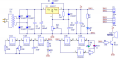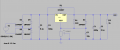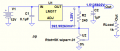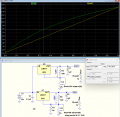# Regulated Power Supply

#### yzumite

Joined May 16, 2023
5
Hello,
I bought a DIY regulated power supply and I wanted to make a simulation for it, the device gives from 1.25V to 12V, but the simulation shows the voltage from 1.4v to 9v what am I doing wrong?
in files official scheme and my LTspice#### crutschow

Joined Mar 14, 2008
33,363
what am I doing wrong?
Your source sinewave is 12Vpk not 12Vrms, so the maximum rectified DC voltage is about 10.8V.
(For 12Vrms, set the sine voltage to 12*√2 = 17Vpk).
The minimum voltage drop across the LM317 is about 2V, giving a maximum output of about 8.8V.
You simulated 9V, so that's about right.

If the pot wiper is all the way to the top, then the output should be 1.25V.

Last edited:
•MrAl and yzumite

#### Papabravo

Joined Feb 24, 2006
20,610
It is not clear from the screenshot how you are changing the value of X1, the potentiometer subcircuit.

#### yzumite

Joined May 16, 2023
5
i change Val from 1 to 99, i did it from this tutorial :

#### Attachments

• 3.2 KB Views: 1

#### crutschow

Joined Mar 14, 2008
33,363
i change Val from 1 to 99, i did it from this tutorial :
Try changing it from 0 to 100.

#### yzumite

Joined May 16, 2023
5
still same, i change potentiometer to resistor 5k and still it gives 9 volts on out

#### crutschow

Joined Mar 14, 2008
33,363
still same, i change potentiometer to resistor 5k and still it gives 9 volts on out

#### yzumite

Joined May 16, 2023
5
Sorry, I have not seen that, after change sin amplitude it shows 1,6 v / 13,50

#### crutschow

Joined Mar 14, 2008
33,363
after change sin amplitude it shows 1,6 v / 13,50
If you put a short across the pot, the output should be 1.25V.

#### MrAl

Joined Jun 17, 2014
10,914
Sorry, I have not seen that, after change sin amplitude it shows 1,6 v / 13,50

Look at the peak output of the transformer. It should be about 17v peak, 34v peak to peak.
Potentiometers do not always go to zero Ohms at the end of travel. Try shorting out the pot to read the low end voltage output.

#### crutschow

Joined Mar 14, 2008
33,363
The LM317 maintains a constant 1.25V between the OUT pin and the ADJ pin, thus the (constant) current through R1 is 1.25 / 240 = 5.21mA.
This current then flows through the set value of the pot to generate the output voltage.
If the pot is set to 1% of its resistance, then the voltage across the pot is 5.21mA * 50Ω = 0.260V.
The minimum output voltage is then 1.25V + 0.260V = 1.51V, close to what you simulated.

My sim:Last edited:
•yzumite

#### yzumite

Joined May 16, 2023
5
Thank you all#### MrAl

Joined Jun 17, 2014
10,914
Thank you allHi,

You're welcome, and also, for the top end and following the logic used in post #11, if you have 5ma through a 5k resistor you get 25v, and adding another 1.24v to that you get a top end voltage of 26.4v if you had a high enough input, but since you are going for just 12v output you could change the pot to a 2.5k value and get closer to what you need. You could put a 5k resistor in parallel to the 5k pot also, which will give you the max of 2.5k, although the voltage adjustment will not be perfectly linear with the pot knob rotation angle using a regular linear taper pot. It probably won't be too noticeable though as the resistance with pot rotation angle will be only mildly nonlinear.
If you don't change the pot value in some way you will only get an adjustment range that spans only about 1/2 of the entire rotation of the pot, meaning turning the pot past about 50 percent in one direction will do nothing, and it works nicer if you use the entire pot rotation range.

#### crutschow

Joined Mar 14, 2008
33,363
going for just 12v output you could change the pot to a 2.5k value and get closer to what you need. You could put a 5k resistor in parallel to the 5k pot also, which will give you the max of 2.5k, although the voltage adjustment will not be perfectly linear with the pot knob rotation angle using a regular linear taper pot. It probably won't be too noticeable though as the resistance with pot rotation angle will be only mildly nonlinear.
Below is a sim that compares the two options, showing the difference in linearity:
The 5k linear pot with a 5k resistor across it outputs 1/2 the maximum voltage (green trace) at the 33% pot rotation point (horizontal axis) instead of 50% for the 2.5k linear pot (yellow trace).Last edited:

#### MisterBill2

Joined Jan 23, 2018
16,612
Understand what is stated in post #2: the reality is that you can not get more voltage out of a series regulator than you put in to it. So go back to the circuit in post #1 and measure the voltage, relative to the common, at the positive terminal of capacitor C3.Then subtract the munimum voltage drop of the regulator IC. That will explain it all.
And I started this reply before the other explanations were posted.

#### MrAl

Joined Jun 17, 2014
10,914
Below is a sim that compares the two options, showing the difference in linearity:
The 5k linear pot with a 5k resistor across it outputs 1/2 the maximum voltage (green trace) at the 33% pot rotation point (horizontal axis) instead of 50% for the 2.5k linear pot (yellow trace).

View attachment 294382

Hi there,

Yes, there will be some nonlinearity.
Up to about 41 percent of full rotation the voltage adjustment will be slightly more touchy, after 41 percent it will be a more fine adjustment.
With a 5k pot alone and no resistor though we loose 50 percent of the entire total rotation.

The following plots show the different ways of looking at it (the diagram with the 6 plot lines).
We can see the problem with just a 5k pot where we only get use out of 50 percent of the rotation. The range starts at 0 and goes to point F, or 1.2v to point F which would then be about 12.5 volts.
We can also see how linear the adjustment vs output is with the 2.5k alone.
We can see that if we dont have a 2.5k pot then the next best thing is to connect a 5k resistor in parallel to the 5k pot. The 5k resistor has to be connected from the arm terminal to one end of the pot though, which would be normal if the arm was shorted to one end anyway in the form of a rheostat. There is another configuration for the fixed resistor but the result is a much more nonlinear response which would only be good for special cases where we need very fine adjustment on one end of the adjustment range and more coarse adjustment at the other end.

BTW all the pots in the illustrations assume the pots are the linear taper type not the audio taper type. Might be interesting to look at the audio taper type possibilities too.
Also note i did not add the 1.24v to the entire output voltage for the plots. That would only be possible with a negative bias of 1.24 volts.

In the second plot (just two plot lines) the 2.5k pot does not cover the entire range so we loose about 10 percent of the rotation travel. Everything over the violet line is lost. We'd have to add a parallel resistor to that also for better results.

Last edited:

#### Audioguru again

Joined Oct 21, 2019
6,451
An LM317 simulation uses only "typical" specs. But if you build the circuit, some devices have a minimum load current of 10mA, not 5mA like a more expensive LM117. Then your output voltage will be too high until the total load current (including the current in the 240 ohms resistor and pot) are 10mA. Use 120 ohms to replace the 240 ohms then the unloaded current is 1.25V/120 ohms= 10.4mA and all LM317 devices will work properly.

Since you want a maximum adjusted voltage of 12V then the pot resistance must be reduced to (12V - 1.25V)/10.4mA= 1034 ohms.

#### MrAl

Joined Jun 17, 2014
10,914
An LM317 simulation uses only "typical" specs. But if you build the circuit, some devices have a minimum load current of 10mA, not 5mA like a more expensive LM117. Then your output voltage will be too high until the total load current (including the current in the 240 ohms resistor and pot) are 10mA. Use 120 ohms to replace the 240 ohms then the unloaded current is 1.25V/120 ohms= 10.4mA and all LM317 devices will work properly.

Since you want a maximum adjusted voltage of 12V then the pot resistance must be reduced to (12V - 1.25V)/10.4mA= 1034 ohms.
Hello there,

Well, in the case of 120 Ohms for that 'feedback' resistor, i would have to recommend using 115 Ohms instead. That way the output is adjustable with a 1k pot up to 12 volts. To get up to 12.5 volts, i would recommend using 110 Ohms. There will be a tiny bit more voltage (some 20mv or so extra) but that should be ignored.

The formula i use is:
Vout=(1.24*(R1+dR1)*R2)/(Rs*(R2+R1+dR1))+1.24
where
1.24v is the internal reference voltage and you can change all occurrences of that to 1.25 if you prefer,
R1 is the pot resistance, varies from 0 to max,
Rs is the current set resistor (120, 115, or 110 Ohms here),
dR1 is any series resistance placed in series with the pot, which gets us up to a higher voltage at the expense of a higher min voltage also,
and if there is no series resistance then dR1=0,
R2 is a parallel resistance, in parallel to the pot, and if there is none then we have instead:
Vout=(1.24*R1+1.24*dR1+1.24*Rs)/Rs

Anyway, lowering the 120 Ohms a little gives us a slightly higher output voltage without raising the min from 1.24 as that stays the same while allowing us to use a 1k pot with no parallel resistor.

It might be a little interesting to note that if we could use a constant current current sink on the output, we could use higher value resistors for Rs and for the pot too. That of course would make the circuit a little more complicated.
The more usual mods are to add a negative bias of around 1.24v so we can adjust all the way to zero volts on the output, and also to add a little current limit adjustment which for a non precision implementation only requires a transistor and a couple resistors and a pot.

#### Bordodynov

Joined May 20, 2015
3,117

#### MrAl

Joined Jun 17, 2014
10,914
Hi,

I think it would be good if you could mention what it is you are trying to show with this simulation.

•MisterBill2